## Friday, 8 August 2014

### CHAPTER 20 -Cross Product

The dot product discussed in the previous section, was introduced through the requirement that arose in calculating the work done by a given force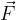$\vec F$ when the point of application of the force is displaced by a certain amount given by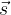$\vec s$: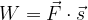$W = \vec F \cdot \vec s$
In this section, we’ll see that another form of vector product exists and is extremely useful to discuss many different physical phenomena; this product is called the cross product. The cross product of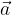$\vec a$ and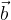$\vec b$ is another vector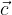$\vec c$ and the relation is represented as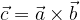$\vec c = \vec a \times \vec b$
Let us, through a physical example, understand what the cross product means.
Consider a horizontal magnetic field, which we can represent by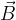$\vec B$, and a charge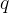$q$ projected into this field with a velocity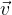$\vec v$ (at an angle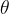$\theta$ with the horizontal).Experiments show that the force$\vec F$ acting on this particle
(a) is perpendicular to the plane of$\vec v$ and$\vec B$ and goes into the plane for the figure above.
(b) increases with increase in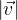$\left| {\vec v} \right|$ and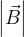$\;\left| {\vec B} \right|$.
(c) is such that its magnitude increases as$\theta$ goes from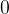$0$ to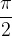$\dfrac{\pi }{2}$ . In fact, when$\vec v$and$\vec B$ are parallel, the force on the particle is zero. For fixed magnitudes of$\vec v$and$\vec B$, the force is the maximum when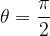$\theta = \dfrac{\pi }{2}$.
(d) increases with increase in charge.
This suggests the dependence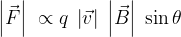$\left| {\vec F} \right|\; \propto q\;\left| {\vec v} \right|\;\left| {\vec B} \right|\;\sin \theta$
which has been confirmed experimentally. In fact, the relation is (exactly),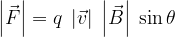$\left| {\vec F} \right| = q\;\left| {\vec v} \right|\;\left| {\vec B} \right|\;\sin \theta$
The direction of$\vec F$ is found out to satisfy the right hand thumb rule. Holding out your thumb use your right hand fingers to map out the rotation from$\vec v$ to$\vec B$. The direction of$\vec F$ is given by the direction in which the thumb points.
Now, since$\vec F$ is a vector with direction perpendicular to both$\vec v$ and$\vec B$, we write the expression for$\vec F$ as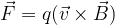${\vec F = q(\vec v \times \vec B)}$
where the vector$\vec v \times \vec B$, the cross product of$\vec v$ and$\vec B$, is understood to be a vector such that its magnitude is$\left| {\vec v} \right|\left| {\vec B} \right|\sin \theta .$ and its direction is given by the right hand thumb rule
In general, the cross product of$\vec a$ and$\vec b$, i.e.$\vec c = \vec a \times \vec b$ is a vector with magnitude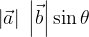$\left| {\vec a} \right|\;\left| {\vec b} \right|\sin {\rm{\theta }}$ (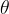${\rm{\theta }}$ being the angle between$\vec a$ and$\vec b$) and direction perpendicular to the plane of$\vec a$ and$\vec b$ such that$\vec a$$\vec b$ and this direction form a right handed system.It is important to keep in mind that the cross product is a vector; the dot product was a scalar. The cross product is also referred to as the vector product.
The cross product of$\vec a$ and$\vec b$, say$\vec c$, has an interesting geometrical interpretation. Since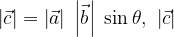$\left| {\vec c} \right| = \left| {\vec a} \right|\;\left| {\vec b} \right|\;\sin \theta ,\,\,\left| {\vec c} \right|$ represents the area of the parallelogram with adjacent sides$\vec a$ and$\vec b$:In fact, the area of the parallelogram can itself be treated as a vector (as it is in physical phenomena):$\vec A = \vec a \times \vec b$
The area of the triangle formed with$\vec a$ and$\vec b$ as two sides is simply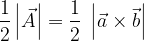$\dfrac{1}{2}\left| {\vec A} \right| = \dfrac{1}{2}\;\left| {\vec a \times \vec b} \right|$.